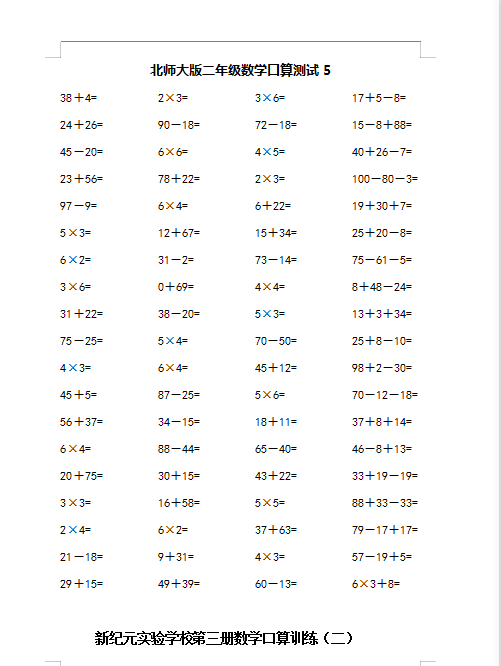38＋4=          2×3=           3×6=           17＋5－8=

24＋26=         90－18=         72－18=         15－8＋88=

45－20=         6×6=           4×5=           40＋26－7=

23＋56=         78＋22=         2×3=           100－80－3=

97－9=          6×4=           6＋22=          19＋30＋7=

5×3=           12＋67=         15＋34=         25＋20－8=

6×2=           31－2=          73－14=         75－61－5=

3×6=           0＋69=          4×4=           8＋48－24=

2022：新北师大版小学数学2年级下册口算 (6)（doc文档4电页子档下载）